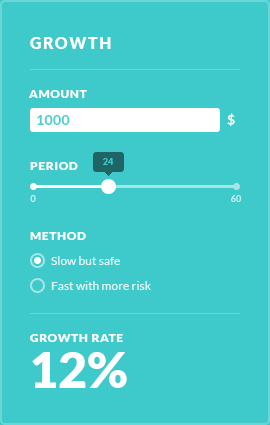# Calorie calculator

The calorie calculator can help you determining how many calories should you need to eat in a day and how many to cut if you want to lose weight.

### Formula

• #### Daily calories needed to maintain your weight:

((10* Weight *0.45359237)+(6.25*(Height *12+#9)*2.54) - (5* Age)+ Gender)* Activity

• #### Daily calories needed to lose 1 lb per week:

(((10* Weight *0.45359237)+(6.25*(Height *12+#9)*2.54) - (5* Age)+ Gender)* Activity)-500

• #### Daily calories needed to lose 2 lb per week:

(((10* Weight *0.45359237)+(6.25*(Height *12+#9)*2.54) - (5* Age)+ Gender)* Activity)-1000

### Works best for

The calories calculator finds out how many calories a day you need, and calculate how many need to lose weigh.